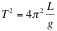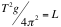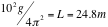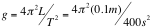Pendulum Problems

Examples using Huygen’s Law of for the period of a Pendulum. using the period, T of a pendulum depends on the square root of L, the length of the pendulum and g, the acceleration due to gravity.Additionally, the frequency f, and the period T, are reciprocals.    T = 1/f

Although we could use any unit for the period (years, months, eons, etc) the standard metric unit is the second.

The metric unit for frequency is Hertz which is one cycle per second.  However, another common units is rpm (rotations per minute).

Example 1:

What is the frequency of a pendulum with a length of 24″?

Unknown: f = ???

First, convert the length into meters

L = 24″ =60.96 cm = 0.610 m

Next, plug the length and acceleration due to gravity into Huygen’s Law.

T = 2π √(.610/9.80) = 2π (0.2495) s = 1.57 s

Now that we have the period T, we take the reciprocal to find the frequency.

f = 1/T = 1/1.57 s = 0.647 Hz

Example 2:

What is the length of a pendulum with a period of 10 seconds?

Unknown: L = ???

First, we take Huygen’s Law:and we algebraically isolate the variable L.  First we square both sides of the equation.Then cross-multiply to isolate LThen we plug in our values for T and g.Example 3:

Suppose you are an astronaut and need to find the acceleration due to gravity on an asteroid using a pendulum.  The period of the pendulum is 20 seconds and the length of the pendulum is 10 cm.  What is g?

Givens: L = 10 cm = 0.1 mUnknown: T = ???

First, we take Huygen’s Law:and we algebraically isolate the variable L.  First we square both sides of the equation.Then cross-multiply to isolate gresulting in an acceleration of gravity of about

g = 0.01 m/s2